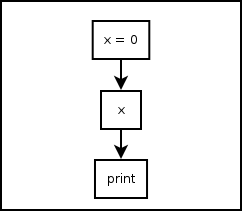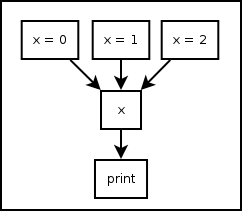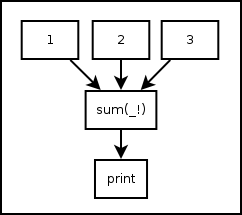# 4. Variables¶

## 4.1. Assignment Statement¶

Pythonect supports standard Python types, but with a twist!

### 4.1.1. Variable `<slug>` in Single Thread¶

You can define a variable and set its value as follows:

```[x = 0] -> x -> print
```

Graphically (Visual programming wise), this is represented as:Both versions print `0`. You can also change its value during the program runtime as follows:

```[x = 0] -> x -> print -> [x = 1] -> x -> print
```

This will print `0` and `1`, in this order, and in the same thread. Of course you can assign and re-assign any legal Python value, for example:

```[x = 0] -> x -> print -> [x = "Hello, world"] -> x -> print
```

Will print `0`, and `"Hello, world"`

### 4.1.2. Variable `<slug>` in Multithreading¶

You can define a variable and set it with multiple values at the same time (each value `map()`‘ed to a different thread) as follows:

```[x = 0, x = 1, x = 2] -> x -> print
```

Graphically (Visual programming wise), this is represented as:Both versions will print, each in its own thread, and not necessarily in that order: `0`, `1`, `2`.

Of course you can assign any combinations of Python value and types, for example:

```[x = 1, x = 1, x = 0.34, x = "Hello, world"] -> x -> print
```

This will print, each in its own thread, and not necessarily in that order: `1`, `1`, `0.34`, and `"Hello world"`.

## 4.2. Predefined Variables¶

Pythonect predefines two variables: `_` and `_!`

### 4.2.1. `_` as Current Value¶

The variable underscore (i.e. `_`) is predefined to be the current value on the flow, for example:

```1 -> _ + 1 -> print
```

Will print `2`. As `_` will be equal `1` after evaluating the `1` expression. Another example is:

```["To be", "Not to be"] -> print "To be, or not to be? " + _
```

This will print, each in its own thread, and not necessarily in that order: `To be, or not to be? To be` and `To be, or not to be? Not to be`

### 4.2.2. `_!` as All Current Values¶

The variable underscore question mark (i.e. `_!`) is predefined to be the list of all the current values in the program (i.e. `reduce()`‘ed), for example:

```[1,2,3] -> sum(_!) -> print
```

Graphically (Visual programming wise), this is represented as:Both versions will print `6`. Notice how `sum` became a reduce-like function, when it accepted a list of `1`, `2`, `3` and returned `6`. Another example:

```"Hello, world" -> reversed -> reduce(lambda x,y: x+y, _!) -> print
```

This will print `"dlrow ,olleH"` (i.e. `"Hello, world"` reversed)